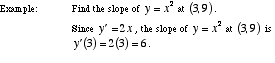index: click on a letter A B C D E F G H I J K L M N O P Q R S T U V W X Y Z A to Z index index: subject areas numbers & symbols sets, logic, proofs geometry algebra trigonometry advanced algebra & pre-calculus calculus advanced topics probability & statistics real world applications multimedia entrieswww.mathwords.com about mathwords website feedback

 Slope of a Curve A number which is used to indicate the steepness of a curve at a particular point. The slope of a curve at a point is defined to be the slope of the tangent line. Thus the slope of a curve at a point is found using the derivative.